# Math in Focus Grade 1 Chapter 16 Practice 3 Answer Key Comparing, Ordering, and Patterns

Go through the Math in Focus Grade 1 Workbook Answer Key Chapter 16 Practice 3 Comparing, Ordering, and Patterns to finish your assignments.

## Math in Focus Grade 1 Chapter 16 Practice 3 Answer Key Comparing, Ordering, and Patterns

Find the missing numbers.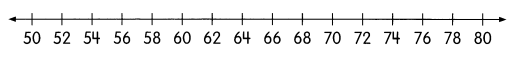Example

2 more than 50 is 52.
2 less than 66 is 64.

Question 1.
2 more than 54 is ____________.
2 more than 54 is 56.

Explanation:
Given that 2 more than 54 which is 54+2 = 56.

Question 2.
______________ is 2 more than 66.
68 is 2 more than 66.

Explanation:
Given that 2 more than 66 which is 66+2 = 68.

Question 3.
2 less than 78 is ______________.
2 less than 78 is 76.

Explanation:
Given that 2 less than 78 which is 78-2 = 76.

Question 4.
____________ is 2 less than 74.
72 is 2 less than 74.

Explanation:
Given that 2 less than 74 which is 74-2 = 72.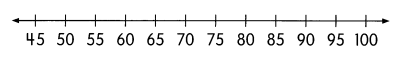Question 5.
5 more than 50 is ______________.
5 more than 50 is 55.

Explanation:
Given that 5 more than 50 which is 50+5 = 55.

Question 6.
10 more than 85 is ______________.
10 more than 85 is 95.

Explanation:
Given that 10 more than 85 which is 95.

Question 7.
5 less than 65 is ______________.
5 less than 65 is 60.

Explanation:
Given that 5 less than 65 which is 65-5 = 60.

Question 8.
____________ is 10 less than 100.
10 less than 100 is 90.

Explanation:
Given that 10 less than 100 which is 100-10 = 90.

Question 9.
____________ is 5 more than 75.
5 more than 75 is 80.

Explanation:
Given that 5 more than 75 which is 80.

Question 10.
____________ is 5 less than 75.
5 less than 75 is 70.

Explanation:
Given that 5 is less than 75 which is 70.

Circle the greatest number.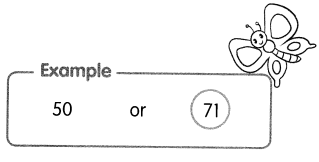Question 11.
72 or 87
87.

Explanation:
Given numbers are 72 and 87. So the greatest number is 87.

Question 12.
92 or 69
92.

Explanation:
Given numbers are 92 and 69. So the greatest number is 92.

Question 13.
54 or 45
54.

Explanation:
Given numbers are 54 and 45. So the greatest number is 54.

Question 14.
67 or 76
76.

Explanation:
Given numbers are 67 and 76. So the greatest number is 76.

Question 15.
86 or 83
86.

Explanation:
Given numbers are 86 and 83. So the greatest number is 86.

Question 16.
94 or 98
98.

Explanation:
Given numbers are 94 and 98. So the greatest number is 98.

Color the number that is less.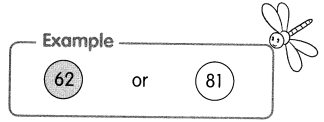Question 17.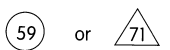59 is less than 71.

Explanation:
Given numbers are 59 and 71. The number 59 is less than 71.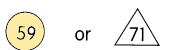Question 18.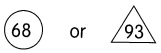68 is less than 93.

Explanation:
Given numbers are 68 and 93. The number 68 is less than 93.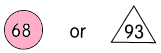Question 19.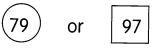79 is less than 97.

Explanation:
Given numbers are 79 and 97. The number 79 is less than 97.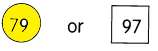Question 20.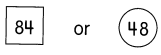48 is less than 48.

Explanation:
Given numbers are 84 and 48. The number 48 is less than 84.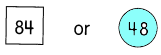Question 21.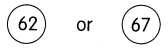62 is less than 67.

Explanation:
Given numbers are 62 and 67. The number 62 is less than 67.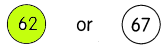Question 22.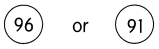Explanation:
Given numbers are 96 and 91. The number 91 is less than 96.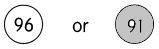Compare the numbers. Then fill in the blanks.

Question 23.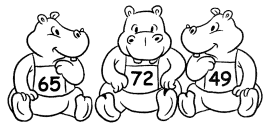The least number is ___________.
The greatest number is __________.
The least number is 49.
The greatest number is 72.

Explanation:
In the above image, the least number is 49 and the greatest number is 72.

Question 24.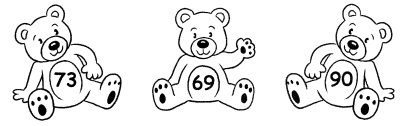The least number is ___________.
The greatest number is __________.
The least number is 69.
The greatest number is 90.

Explanation:
In the above image, the least number is 69 and the greatest number is 90.

Question 25.
Order the numbers from greatest to least.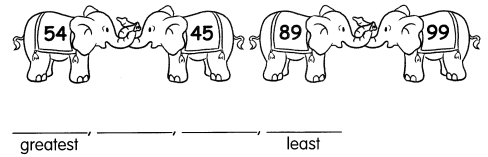99,89,54,45.

Explanation:
The numbers from greatest to least is 99,89,54,45.

Use the numbers to fill in the blanks.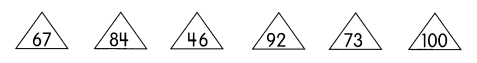Question 26.
The greatest number is __________.
100.

Explanation:
The greatest number is 100.

Question 27.
The least number is __________.
46.

Explanation:
The least number is 46.

Question 28.
__________, __________, and ________ are less than 84.
46,67 and 73.

Explanation:
46,63 and 73 are less than 84.

Question 29.
____________ and __________ are greater than 84.
92,100.

Explanation:
92 and 100 are greater than 84.

Question 30.
67 is greater than ___________ but less than 100.
46.

Explanation:
67 is greater than 46 but less than 100.

Question 31.
92 is less than ___________ but greater than 84.
100.

Explanation:
92 is less than 100 but greater than 84.

Complete each number pattern.

Question 32.
50, 51, 52, __________, 54, 55, __________, __________, 58
50,51,52,53,54,55,56,57,58.

Explanation:
The complete pattern is 50,51,52,53,54,55,56,57,58.

Question 33.
73, 72, 71, __________, __________, 68, __________
73,72,71,70,69,68,67.

Explanation:
The complete pattern is 73,72,71,70,69,68,67.

Question 34.
__________, 87, 89, __________, 93, __________
85,87,89,91,93,95.

Explanation:
The complete pattern is 85,87,89,91,93,95.

Question 35.
99, __________, 95, 93, __________, __________

Question 36.
50, 60, __________, 80, __________, __________